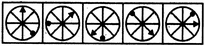# Non Verbal Reasoning - Classification - Discussion

### Discussion :: Classification - Section 2 (Q.No.1)

In each problem, out of the five figures marked (1), (2), (3), (4) and (5), four are similar in a certain manner. However, one figure is not like the other four. Choose the figure which is different from the rest.

1.

Choose the figure which is different from the rest.(1)     (2)     (3)     (4)     (5)

 [A]. 1 [B]. 2 [C]. 3 [D]. 4 [E]. 5

Explanation:

Only in fig. (4), the black triangle and the black circle lie at the two ends of the same diameter.

 Praneeth said: (Jul 21, 2015) 4th one is the answer because the two symbols are on either side of the diameter.

 Nantanka said: (Dec 4, 2016) The fourth one because it makes the diameter.

 Shiladitya said: (Jun 21, 2017) Diagram C also same like 5 then why 5 is the answer?